Search by Topic

Resources tagged with Mathematical modelling similar to Random Squares:

Filter by: Content type:
Age range:
Challenge level:

There are 72 results

Broad Topics > Using, Applying and Reasoning about Mathematics > Mathematical modellingTime to Evolve 2

Age 16 to 18 Challenge Level:

How is the length of time between the birth of an animal and the birth of its great great ... great grandparent distributed?Population Dynamics - Part 2

Age 16 to 18 Challenge Level:

Second in our series of problems on population dynamics for advanced students.Pdf Stories

Age 16 to 18 Challenge Level:

Invent scenarios which would give rise to these probability density functions.FA Cup

Age 16 to 18 Challenge Level:

In four years 2001 to 2004 Arsenal have been drawn against Chelsea in the FA cup and have beaten Chelsea every time. What was the probability of this? Lots of fractions in the calculations!Population Dynamics - Part 1

Age 16 to 18 Challenge Level:

First in our series of problems on population dynamics for advanced students.Population Dynamics - Part 3

Age 16 to 18 Challenge Level:

Third in our series of problems on population dynamics for advanced students.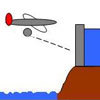Dam Busters 1

Age 16 to 18 Challenge Level:

At what positions and speeds can the bomb be dropped to destroy the dam?Population Dynamics - Part 4

Age 16 to 18 Challenge Level:

Fourth in our series of problems on population dynamics for advanced students.Population Dynamics - Part 5

Age 16 to 18 Challenge Level:

Fifth in our series of problems on population dynamics for advanced students.Population Dynamics - Part 6

Age 16 to 18 Challenge Level:

Sixth in our series of problems on population dynamics for advanced students.The Mean Game

Age 16 to 18

Edward Wallace based his A Level Statistics Project on The Mean Game. Each picks 2 numbers. The winner is the player who picks a number closest to the mean of all the numbers picked.Population Ecology Using Probability

Age 16 to 18 Challenge Level:

An advanced mathematical exploration supporting our series of articles on population dynamics for advanced students.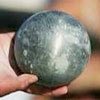Model Solutions

Age 16 to 18 Challenge Level:

How do these modelling assumption affect the solutions?Over-booking

Age 16 to 18 Challenge Level:

The probability that a passenger books a flight and does not turn up is 0.05. For an aeroplane with 400 seats how many tickets can be sold so that only 1% of flights are over-booked?Population Dynamics Collection

Age 16 to 18 Challenge Level:

This is our collection of tasks on the mathematical theme of 'Population Dynamics' for advanced students and those interested in mathematical modelling.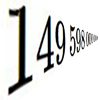Big and Small Numbers in Physics - Group Task

Age 16 to 18 Challenge Level:

Work in groups to try to create the best approximations to these physical quantities.Ramping it Up

Age 16 to 18 Challenge Level:

Look at the calculus behind the simple act of a car going over a step.Age 16 to 18 Challenge Level:

This is the section of stemNRICH devoted to the advanced applied mathematics underlying the study of the sciences at higher levels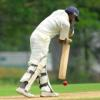Twenty20

Age 7 to 16 Challenge Level:

Fancy a game of cricket? Here is a mathematical version you can play indoors without breaking any windows.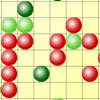Epidemic Modelling

Age 14 to 18 Challenge Level:

Use the computer to model an epidemic. Try out public health policies to control the spread of the epidemic, to minimise the number of sick days and deaths.An Introduction to Computer Programming and Mathematics

Age 16 to 18

This article explains the concepts involved in scientific mathematical computing. It will be very useful and interesting to anyone interested in computer programming or mathematics.Predator - Prey Systems

Age 16 to 18 Challenge Level:

See how differential equations might be used to make a realistic model of a system containing predators and their prey.Modelling Assumptions in Mechanics

Age 16 to 18

An article demonstrating mathematically how various physical modelling assumptions affect the solution to the seemingly simple problem of the projectile.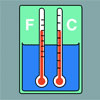Stemnrich - the Physical World

Age 11 to 16 Challenge Level:

PhysNRICH is the area of the StemNRICH site devoted to the mathematics underlying the study of physicsBionrich

Age 14 to 18 Challenge Level:

bioNRICH is the area of the stemNRICH site devoted to the mathematics underlying the study of the biological sciences, designed to help develop the mathematics required to get the most from your. . . .Physnrich

Age 14 to 18 Challenge Level:

PhysNRICH is the area of the StemNRICH site devoted to the mathematics underlying the study of physicsSpinners

Age 16 to 18 Challenge Level:

How do scores on dice and factors of polynomials relate to each other?Engnrich

Age 16 to 18 Challenge Level:

engNRICH is the area of the stemNRICH Advanced site devoted to the mathematics underlying the study of engineeringThe Legacy

Age 14 to 16 Challenge Level:

Your school has been left a million pounds in the will of an ex- pupil. What model of investment and spending would you use in order to ensure the best return on the money?Spot the Card

Age 14 to 16 Challenge Level:

It is possible to identify a particular card out of a pack of 15 with the use of some mathematical reasoning. What is this reasoning and can it be applied to other numbers of cards?The Wrong Stats

Age 16 to 18 Challenge Level:

Why MUST these statistical statements probably be at least a little bit wrong?Where to Land

Age 14 to 16 Challenge Level:

Chris is enjoying a swim but needs to get back for lunch. If she can swim at 3 m/s and run at 7m/sec, how far along the bank should she land in order to get back as quickly as possible?In Constantly Passing

Age 14 to 16 Challenge Level:

A car is travelling along a dual carriageway at constant speed. Every 3 minutes a bus passes going in the opposite direction, while every 6 minutes a bus passes the car travelling in the same. . . .Population Dynamics

Age 16 to 18 Challenge Level:

This problem opens a major sequence of activities on the mathematics of population dynamics for advanced students.Escalator

Age 14 to 16 Challenge Level:

At Holborn underground station there is a very long escalator. Two people are in a hurry and so climb the escalator as it is moving upwards, thus adding their speed to that of the moving steps. . . .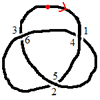Drawing Doodles and Naming Knots

Age 7 to 18

This article for students introduces the idea of naming knots using numbers. You'll need some paper and something to write with handy!What's a Knot?

Age 7 to 16 Challenge Level:

A brief video explaining the idea of a mathematical knot.Designing Table Mats

Age 11 to 16 Challenge Level:

Formulate and investigate a simple mathematical model for the design of a table mat.Investigating Epidemics

Age 11 to 16 Challenge Level:

Simple models which help us to investigate how epidemics grow and die out.Guessing the Graph

Age 14 to 16 Challenge Level:

Can you suggest a curve to fit some experimental data? Can you work out where the data might have come from?Snooker

Age 16 to 18 Challenge Level:

A player has probability 0.4 of winning a single game. What is his probability of winning a 'best of 15 games' tournament?Fixing the Odds

Age 14 to 16 Challenge Level:

You have two bags, four red balls and four white balls. You must put all the balls in the bags although you are allowed to have one bag empty. How should you distribute the balls between the two. . . .
Concrete Calculation

Age 14 to 16 Challenge Level:

The builders have dug a hole in the ground to be filled with concrete for the foundations of our garage. How many cubic metres of ready-mix concrete should the builders order to fill this hole to. . . .Branching Processes and Extinction

Age 16 to 18 Challenge Level:

An advanced mathematical exploration supporting our series of articles on population dynamics for advanced students.Shaping the Universe III - to Infinity and Beyond

Age 11 to 16

The third installment in our series on the shape of astronomical systems, this article explores galaxies and the universe beyond our solar system.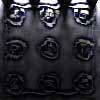Scratch Cards

Age 14 to 16 Challenge Level:

To win on a scratch card you have to uncover three numbers that add up to more than fifteen. What is the probability of winning a prize?Logic, Truth Tables and Switching Circuits

Age 11 to 18

Learn about the link between logical arguments and electronic circuits. Investigate the logical connectives by making and testing your own circuits and record your findings in truth tables.Chocolate 2010

Age 14 to 16 Challenge Level:

First of all, pick the number of times a week that you would like to eat chocolate. Multiply this number by 2...Logic, Truth Tables and Switching Circuits Challenge

Age 11 to 18

Learn about the link between logical arguments and electronic circuits. Investigate the logical connectives by making and testing your own circuits and fill in the blanks in truth tables to record. . . .Rocking Chairs, Railway Games and Rayboxes

Age 5 to 18

In this article for teachers, Alan Parr looks at ways that mathematics teaching and learning can start from the useful and interesting things can we do with the subject, including. . . .